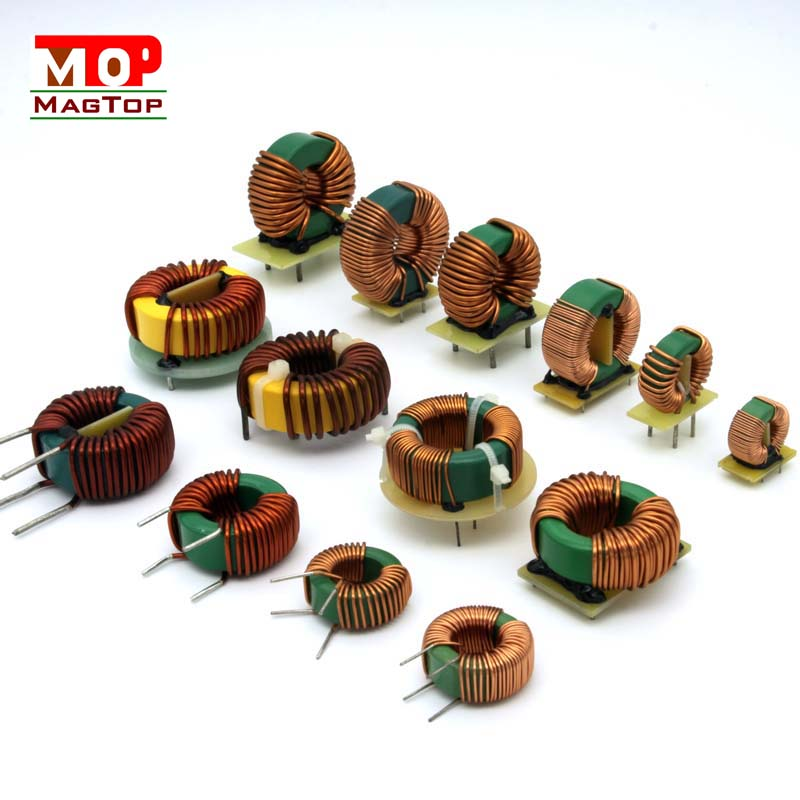# Common inductor’s Parameter calculation procedure

##### author: MagTop
31/03/2023Company News | common mode chokes | Industry News | transformer|RJ45 connectors
Determine the desired common-mode inductor value. This needs to be determined based on the level of common-mode noise in the circuit and the amount of common-mode noise that needs to be suppressed.
Determine the operating frequency. The parameter calculation of common-mode inductance should take into account the effect of operating frequency on inductance value. In general, common-mode inductors should operate at the same frequency as the circuit.
Determine the supply voltage and load resistance. These parameters affect the current and power of common-mode filters
The required common mode inductance parameters are calculated according to the above parameters. The following formula can be used to calculate:
L = V / (I * f)
Where L is the inductance value of the common-mode inductor (in hents), V is the supply voltage (in volts), I is the current through the common-mode inductor (in amperes), and f is the operating frequency (in Hertz). This formula applies only to single-turn coil inductance. If the inductor has multiple turns, other formulas need to be used for calculation.